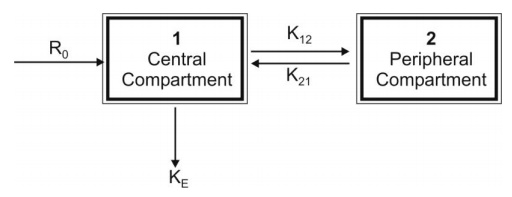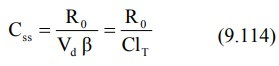# Two-Compartment Open Model: Intravenous Infusion

| Home | | Biopharmaceutics and Pharmacokinetics |

## Chapter: Biopharmaceutics and Pharmacokinetics : Compartment Modelling

The model can be depicted as shown below with elimination from the central compartment.

Two-Compartment Open Model

Intravenous Infusion

The model can be depicted as shown below with elimination from the central compartment.The plasma or central compartment concentration of a drug that fits two-compartment model when administered as constant rate (zero-order) i.v. infusion, is given by equation:At steady-state (i.e. at time infinity), the second and the third term in the bracket becomes zero and the equation reduces to:Now VcKE = Vdß. Substituting this in equation 9.113, we get:The loading dose Xo,L to obtain Css immediately at the start of infusion can be calculated from equation: